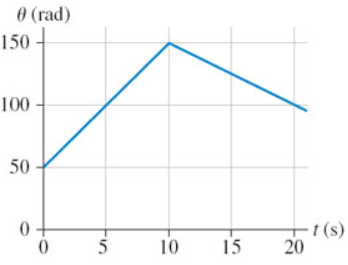# Problem: (a) What is the angular displacement of the wheel between t = 5 s and t = 15 s?(b) What is the angular velocity of the wheel at 15 s?

###### FREE Expert Solution

(a)

Angular displacement is:

$\overline{){\mathbf{∆}}{\mathbf{\theta }}{\mathbf{=}}{{\mathbf{\theta }}}_{{\mathbf{f}}}{\mathbf{-}}{{\mathbf{\theta }}}_{{\mathbf{i}}}}$

90% (187 ratings)###### Problem Details(a) What is the angular displacement of the wheel between t = 5 s and t = 15 s?

(b) What is the angular velocity of the wheel at 15 s?